# Texas Go Math Grade 1 Lesson 5.3 Answer Key Model Taking Apart

Refer to our Texas Go Math Grade 1 Answer Key Pdf to score good marks in the exams. Test yourself by practicing the problems from Texas Go Math Grade 1 Lesson 5.3 Answer Key Model Taking Apart.

## Texas Go Math Grade 1 Lesson 5.3 Answer Key Model Taking Apart

Essential Question
How can you model taking apart, or separating?
Explanation:
Taking apart, or separating represents subtraction.

Explore
Use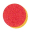to model the problem. Draw and color to show your model. Write the numbers and a subtraction sentence.
Explanation:
4 – 1 = 5Use counters to solve.Explanation: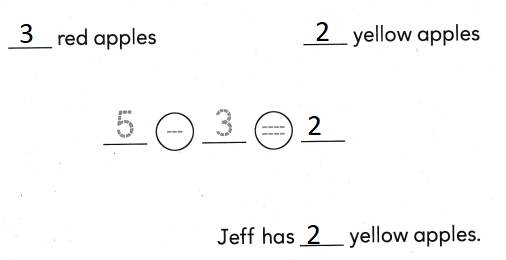For The Teacher

• Have children model the problem using counters. Jeff has 5 apples. 3 apples are red. The rest are yellow. How many apples are yellow?
Explanation:
5 – 3 = 2
so, Jeff had 2 yellow apples

Math Talk
Mathematical Processes
How did you solve this problem? Explain.
Explanation:
We solved the problem by subtracting
from total number of apples – red apples
so, remaining are yellow apples.

Model and Draw
Subtract to find how many small cups there are.

Mary has 6 cups. 2 cups are big. The rest are small. Draw to show how many cups are small.
____ small cups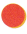Explanation:
4 small cups
6 – 2 = 4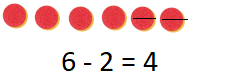Share and Show

Useto solve. Draw to show your work.
Write the number sentence and how many.

Question 1.
There are 7 folders. 6 folders are red. The rest are yellow. How many folders are yellow?
____ yellow folder1 yellow folder
Explanation:
7 – 6 = 1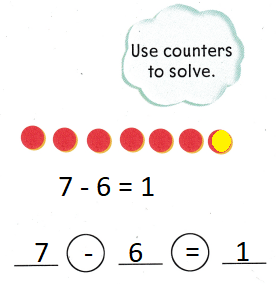Question 2.
There are 8 pencils. 3 pencils are short. The rest are long. How many pencils are long?
___ long pencilsExplanation:
There are 8 pencils.
3 pencils are short.
8 – 3 = 5
5 are long.

Problem Solving

Use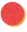to solve. Draw to show your work. Write the number sentence and how many.

Question 3.
There are 9 fish. 5 fish have spots. The rest have stripes. How many fish have stripes?
___ striped fish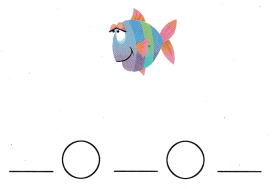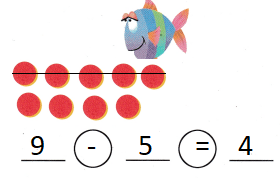4 striped fish
Explanation:
9 – 5 = 4
4 fish have stripes

Write or draw to solve.

Question 4.
There are 6 bears. 4 are big. The rest are small. How many bears are small?____ small bears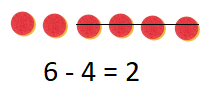Explanation:
There are 6 bears. 4 are big.
6 – 4 = 2
2 are small.

Question 5.
H.O.T. There are 7 bears. 1 bear walks away. Then 4 more bears walk away. How many bears are there now?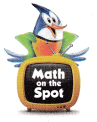___ bears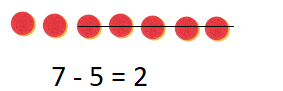Explanation:
1 + 4 = 5
7 – 5 = 2
2 bears are there now

Question 6.
H.O.T. Multi-Step There are 4 bears. Some are black and some are brown. There are fewer than 2 black bears.
How many bears are brown?
____ brown bears
1 brown bears
Explanation:
fewer than 2 black bears means it is 1
3 are brown and 1 is black

Choose the correct answer. Use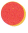to solve.

Question 7.
There are 8 cars on a train. 2 cars are red. The rest are green. How many cars are green?(A) 5
(B) 6
(C) 10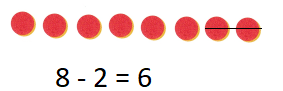Explanation:
8 – 2 = 6
6 cars are green

Question 8.
What number sentence solves the problem? A house has 5 beds. 1 bed is big. The rest are small. How many beds are small?
(A) 5 – 2 = 3
(B) 5 – 1 = 4
(C) 5 + 1 = 6
Explanation:5 – 1 = 4
4 are small beds

Question 9.
Use Tools There are 7 flowers. 3 flowers are yellow. 2 flowers are white. The rest are red. How many flowers are red?
(A) 2
(B) 3
(C) 5Explanation:
3 + 2 = 5
7 – 5 = 2
2 are red

Question 10.
Texas Test Prep Which number sentence solves the problem? There are 4 cups. 3 cups are red. The rest are blue. How many cups are blue?
(A) 4 + 3 = 7
(B) 4 – 2 = 2
(C) 4 – 3 = 1
Explanation:
1 cups is blue
4 – 3 = 1Take Home Activity

• Have your child collect a group of up to 10 small objects of the same kind, and use them to generate subtraction stories.
Explanation:
the  subtraction partners of 10 are
10 – 1 = 9
10 – 2 = 8
10 – 3 = 7
10 – 4 = 6 as on

### Texas Go Math Grade 1 Lesson 5.3 Homework and Practice Answer Key

Draw counters to solve.
Write how many and the number sentence.

Draw counters to solve.
Write how many and the number sentence

Question 1.
There are 8 chairs. 1 chair is big. The rest are small. How many chairs are small?
___ small chairs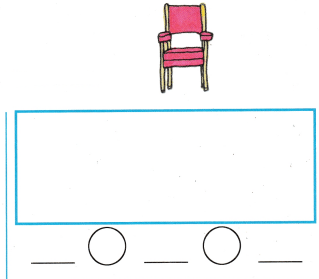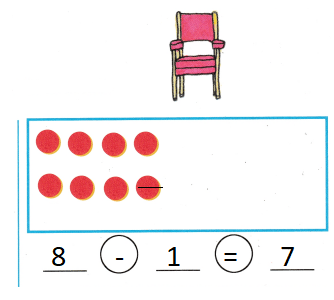Explanation:
8 – 1 = 7
7 chairs are small

Question 2.
There are 7 apples. 3 apples are red. The rest are green. How many apples are green?
____ green apples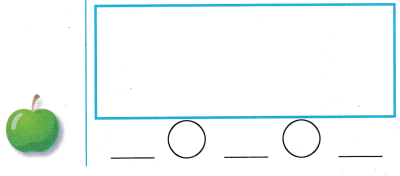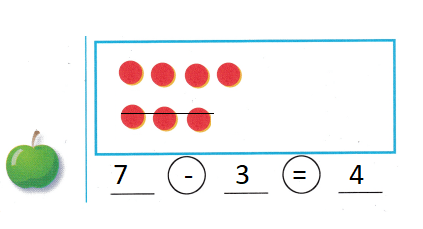Explanation:
There are 7 apples. 3 apples are red.
7 – 3 = 4
4 are green.

Question 3.
A flower has 9 petals. 2 petals fall off. How many petals are there now?
____ petals
7 petals
Explanation:
A flower has 9 petals. 2 petals fall off.
9 – 2 = 7
7 petals are there now

Texas Test Prep

Lesson Check

Question 4.
There are 5 leaves. 4 leaves blow away. How many leaves are there now?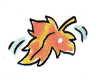(A) 9
(B) 4
(C) 1
Explanation:
5 – 4 = 1
1 leaf is there now

Question 5.
What number sentence solves the problem? A boy has 7 toy cars. 2 cars are blue. The rest are red. How many cars are red?
(A) 7 – 2 = 5
(B) 9 – 2 = 7
(C) 7 + 2 = 9
Explanation:
7 – 2 = 5
A boy has 7 toy cars. 2 cars are blue.
5 are red.

Question 6.
Multi-Step There are 5 parrots. 1 parrot flies away. Then 2 more parrots fly away. How many parrots are there now?
(A) 3
(B) 2
(C) 4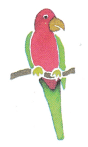Explanation:
1 + 2 = 3
5 – 3 = 2
2 parrots are there now

Question 7.
Which number sentence solves the problem? There are 9 grapes. 3 grapes are green. The rest are purple How many grapes are purple?
(A) 3 + 3 = 6
(B) 9 – 5 = 4
(C) 9 – 3 = 6